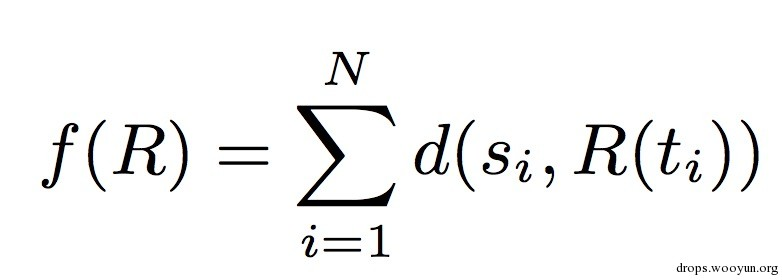# 0x01 思考模式

## 3. 丢一起

``````example ＝ [www.baidu.com, www.google.com, www.hello.com]
``````

``````union = ['www.', '.com']
``````

``````regexPatten = [FullPatten, HalfPatten, FullPatten]
``````

``````re = "www.\w+.com"
``````

# 0x02 数据的多样性

``````#!python
from math import log
def shan(x):
shan = 0.
for i in set(x):
shan -= (float(x.count(i))/len(x))*log(float(x.count(i))/len(x))
return shan

print shan([5,6,5,6])
print shan([5,5,5,5])
``````

out:

``````0.69314718056
0.0
``````

## 1. HalfPatten

``````#!python
class HalfPatten:

def __init__(self,x):
self.x = x
self.type = "halfPatten"
self.shan = 0.
self.regShan= 0.
self.x_length = [len(i) for i in x]
for i in set(self.x_length):
self.shan -= (float(self.x_length.count(i))/len(self.x_length))*log(float(self.x_length.count(i))/len(self.x_length))
for c in set(self.x):
self.regShan -= (float(self.x.count(c))/len(self.x))*log(float(self.x.count(c))/len(self.x))

def detection(self,string):
s = ''.join(string)
if len(s)==0:
return ""
if s.isalnum():
if s.isdigit():
return "\d"
return "\w"
else:
return "."

def gener(self,patten):
if patten=="all":
return "%s+"%(self.detection(self.x))
elif patten=="zone":
x_len = self.x_length
x_len.sort()

return "%s{%i,%i}"%(self.detection(self.x),x_len,x_len[-1])
else:
return "%s{%i}"%(self.detection(self.x),self.x_length)

def regex(self,shanRule):
if self.detection(self.x)=="":
return ""
if self.shan>0:
if self.shan>shanRule:
return self.gener("all")
else:
return self.gener("zone")
else:
return self.gener("length")

def main(shan=1.):
exmple = ['123','123']
exmple2 = ['123','1234']
half = HalfPatten(exmple)
half2 = HalfPatten(exmple2)
print half.regex(shan)
print half2.regex(shan)

if __name__ =="__main__":
main()
main(0.)
``````

out:

``````\d{3}
\d{3,4}
\d{3}
\d+
``````

## 2. FullPatten

fullPatten其实相对于half来说要简单了许多，我们只需要根据确定性的字符串生成对应的正则表达式就好了。需要注意的是所谓的确定性其实也有不同的模式， 比如 我们想匹配 .com 和 .cn结尾的url而不想要其他的东西。现在写成代码：

``````#!python
class FullPatten:

def __init__(self,x):
self.x = x
self.type = "fullPatten"
self.shan = 0.
self.regShan = 0.

def gener(self,patten):
if patten=="all":
return "(%s)"%(self.x)
else:
return "(%s)"%('|'.join(list(set(self.x))))

def regex(self,shanRule):
if len(set(self.x))>1:
return self.gener("half")
else:
return self.gener("all")

def main():
example = ['.fuck','.fuck']
example1 = ['.com','.cn']
full = FullPatten(example)
full1 = FullPatten(example1)
print full.regex(example)
print full1.regex(example1)

if __name__ == "__main__":
main()
``````

out:

``````(.fuck)
(.com|.cn)
``````

## 3. 组合

``````#!python
class strspl:

def __init__(self,y,shan):
self.sentence = []
self.y = y
self.shan = shan

def re_split(self,string):
s = []
for x in self.y:
s.append([x[:x.index(string)],string,x[x.index(string)+len(string):]])
half_1 = []
full_1 = []
half_2 = []
for q,w,e in s:
half_1.append(q)
full_1.append(w)
half_2.append(e)
self.sentence.append(HalfPatten(half_1))
self.sentence.append(FullPatten(full_1))
self.sentence.append(HalfPatten(half_2))

for l,i in enumerate(self.sentence):
if i.shan!=0.:
if i.regShan<self.shan:
self.sentence[l]=FullPatten(i.x)

def main(shan=1.5):
example = ['asb.baidu.go','ww.baidu.com','www.baidu.fuck']
a = strspl(example,shan)
a.re_split('.baidu.')
sentence=[]
regex = []
for i in a.sentence:
sentence.append(i.type)
regex.append(i.regex(0.))
return sentence,''.join(regex)

if __name__ == "__main__":
s,r = main()
print s
print r
s,r = main(0.)
print s
print r
``````

out:

``````['fullPatten', 'fullPatten', 'fullPatten']
(ww|asb|www)(.baidu.)(go|com|fuck)
['halfPatten', 'fullPatten', 'halfPatten']
\w+(.baidu.)\w+
``````

## 4. 丢一起

``````#!python
class Auto:

def __init__(self,x,shan=.3,regShan=.8):
self.x = x
self.sentence = []
self.U = ReU()
self.shan = shan
self.regShan = regShan

def __guess(self,x,y):
if y in x:
return 1
return 0

def generation(self,iter=1):
x = self.x
location = 0.
for i in range(iter):
if len(self.sentence)==0:
str = strspl(self.x,self.regShan)
di = {}
di['data']= x
frame = pd.DataFrame(di)
#这个proccess是我之前写的一个坑爹的模块，其实就是提取共有的字符串，然后key = sorted(x,key=len,reverse=True) return key
key = self.U.proccess(frame,'data')
sen = str.re_split(key)
self.sentence  = str.sentence
continue
print "counting %i"%(i)
chanceShan = 0.
chance = None
for l,i in enumerate(self.sentence):
if i.regShan !=0.:
if chanceShan<i.regShan:
chance = l
chanceShan = i.regShan
if chance!=None:

di={}
di['data']=self.sentence[chance].x
str = strspl(di['data'],self.regShan)
frame = pd.DataFrame(di)
try:
key = self.U.proccess(frame,'data')
except IndexError,e:
break
sen = str.re_split(key)
self.sentence = self.sentence[:chance]+str.sentence+self.sentence[chance+1:]

def build(self):
reg = []
shan = []
for i in self.sentence:
reg.append(i.regex(self.shan))
shan.append(i.regShan)
return shan,"".join(reg)

def main(shan=.7,regShan=.3,it=1):
s = ['www.asb.baids.com','www.ww.baidu.com','www.www.baidu.com']
a = Auto(s,shan,regShan)
a.generation(it)
shan,reg =  a.build()
print shan
print reg

if __name__=="__main__":
main()
main(0,0,)
main(0,1.5)
main(0,1.5,it=2)
main(it=2)
``````

out:

``````[1.0986122886681096, 0.0, 0.6365141682948128]
.{6,7}(.bai).{6}
[1.0986122886681096, 0.0, 0.6365141682948128]
.+(.bai).{6}
[0.0, 0.0, 0.6365141682948128]
(www.ww|www.www|www.asb)(.bai).{6}
counting 1
[0.0, 0.0, 0.6365141682948128, 0.0, 0.0]
(www.ww|www.www|www.asb)(.bai)\w{2}(.com)
counting 1
[0.0, 0.0, 1.0986122886681096, 0.0, 0.6365141682948128]
(www.)\w{2,3}(.bai).{6}
``````

## 5. 选取最佳